# Almost-periodic function on a group

(diff) ← Older revision | Latest revision (diff) | Newer revision → (diff)

A generalization of almost-periodic functions defined on. Letbe an (abstract) group. A bounded complex-valued function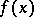,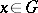, is called a right almost-periodic function if the family, whereruns through the entire group, is (relatively) compact in the topology of uniform convergence on, i.e. if every sequence of functions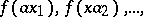contains a subsequence which is uniformly convergent on. A left almost-periodic function onis defined similarly. It turns out that every right (left) almost-periodic functionis also left (right) almost-periodic, and the family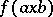, whereandindependently run through, is (relatively) compact. The latter property is often taken as a definition of almost-periodic functions on. The set of all almost-periodic functions onis a Banach space with a norm.

The theory of almost-periodic functions on a group depends essentially on the mean-value theorem (cf. , ). A linear functionaldefined on the space of almost-periodic functions is called a mean value if

1)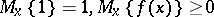for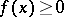andfor,;

2)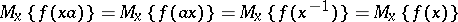, for all.

A unitary matrix function, defined on, is called a unitary representation ofif(is the identity element ofand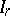is the identity matrix of order) and if for all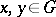,. The numberis called the dimension of the representation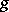. The matrix entries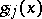are almost-periodic functions on. In the theory of almost-periodic functions on a group they play the same role as the functions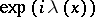in the theory of almost-periodic functions on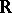.

Two representationsand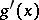are said to be equivalent if a constant matrixexists such that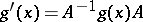. A representationis said to be irreducible if the family of the matrices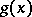,, does not admit a common non-trivial subspace in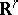. The set of all irreducible unitary representations is partitioned into classes of mutually-equivalent representations. Let one representation be chosen from each equivalence class and let the set thus obtained be denoted by. Then the set of almost-periodic functionsonturns out to be an orthogonal (though, in general, uncountable) system with respect to the mean value.

Theorem 1 (the Parseval equality). For an almost-periodic functionthe following equality holds:(Thus, for only countably many,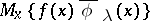differs from zero; the series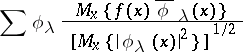is called the Fourier series of.

A representationis said to occur in the Fourier series of an almost-periodic functionif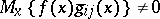for some,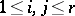.

Theorem 2 (the approximation theorem). The setis dense in the space of almost-periodic functions equipped with the normand every almost-periodic function can be arbitrarily well approximated by a finite linear combination of matrix entries of representations occurring in its Fourier series.

Ifis a topological group, then to the definition of an almost-periodic function should be added the requirement of its continuity. In this case, the representations occurring in its Fourier series are also continuous.

Ifis an Abelian group, then the continuous unitary representations are one-dimensional. They are called the characters of. The characters ofare denoted byand Parseval's equality reads as follows: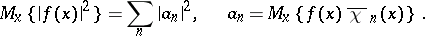In the case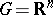the continuous characters are the functions, where,. Theorems 1 and 2 imply the main results in the theory of almost-periodic functions of a single or of several variables.

The proof of the main statements in the theory of almost-periodic functions is based on the consideration of integral equations on a group (cf. ). The existence of sufficiently many linear representations of compact Lie groups has been proved . In this case, invariant integration (and consequently, the mean) can be established directly. Invariant integration on an abstract compact group has been constructed  depending on an extension of the Peter–Weyl theory to this case.

The theory of almost-periodic functions on a group can be deduced (cf. ) from the Peter–Weyl theory in the following way. Letbe an almost-periodic function on a groupand letThen the set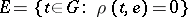is a normal subgroup of,is an invariant metric on the quotient groupandis uniformly continuous on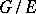.

The almost-periodicity ofimplies that the completion ofin the metricis a compact group and Theorems 1 and 2 follow from the Peter–Weyl theory.